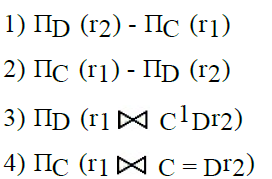# GATE | GATE-CS-2004 | Question 13

Let R1 (A, B, C) and R2 (D, E) be two relation schema, where the primary keys are shown underlined, and let C be a foreign key in R1 referring to R2. Suppose there is no violation of the above referential integrity constraint in the corresponding relation instances r1 and r2. Which one of the following relational algebra expressions would necessarily produce an empty relation ?(A) 1
(B) 2
(C) 3
(D) 4